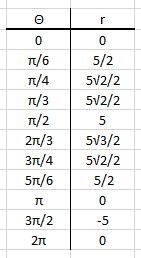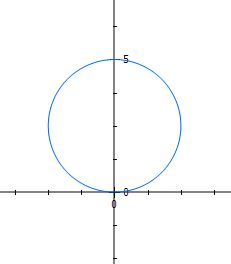# Sketch the curve with the given polar equation. Complete the table of values for the curve over...

## Question:

Sketch the curve with the given polar equation. Complete the table of values for the curve over the interval {eq}0 \leq \theta \leq 2\pi{/eq}.

1. {eq}r = 5{/eq} {eq}sin{/eq} {eq}\theta{/eq}.

## Table of equations:

A table of values is an organizer or chart that helps determine points to plot for a graph. Usually, the header is composed of the {eq}\displaystyle x,y {/eq} coordinates. In a case of a polar equation, however, we will be using {eq}\displaystyle r, \theta {/eq} values.

## Answer and Explanation:

To form the table of values, we simply substitute the value of {eq}\displaystyle \theta {/eq} to the equation. We will get the value of {eq}\displaystyle r {/eq} that way.Below, we can see the graph of {eq}\displaystyle r= 5sin \theta {/eq}#### Learn more about this topic:Graphs: Types, Examples & Functions

from High School Algebra II: Help and Review

Chapter 16 / Lesson 11
355K